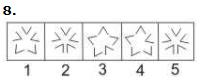Courses

# BITSAT Logical Reasoning Test - 4

## 10 Questions MCQ Test BITSAT Subject Wise & Full Length Mock Tests | BITSAT Logical Reasoning Test - 4

Description
This mock test of BITSAT Logical Reasoning Test - 4 for JEE helps you for every JEE entrance exam. This contains 10 Multiple Choice Questions for JEE BITSAT Logical Reasoning Test - 4 (mcq) to study with solutions a complete question bank. The solved questions answers in this BITSAT Logical Reasoning Test - 4 quiz give you a good mix of easy questions and tough questions. JEE students definitely take this BITSAT Logical Reasoning Test - 4 exercise for a better result in the exam. You can find other BITSAT Logical Reasoning Test - 4 extra questions, long questions & short questions for JEE on EduRev as well by searching above.
QUESTION: 1

Solution:
QUESTION: 2

Solution:
QUESTION: 3

### Group the following figures into three classes on the basis of identical properties.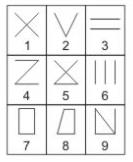Solution:
QUESTION: 4

Find the missing character.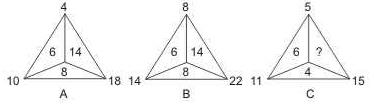Solution:

In fig. (A), 10 - 4 = 6, 18 - 10 = 8, 18 - 4 = 14.
In fig. (B), 14 - 8 = 6, 22 - 14 = 8, 22 - 8 = 14.
In fig. (C), 11 - 5 = 6, 15 - 11 = 4.
So, missing number = 15 - 5 = 10.

QUESTION: 5

In this letter series, some of the letters are missing. Choose the correct letter given below -
a _ bccb _ ca _ cca _ baab _ c

Solution:
QUESTION: 6

In this letter series, some of the letters are missing. Choose the correct letter given below -
aa _ ab _ _ aaa _ a

Solution: It is following some definite pattern ie. (aaaa)(b)(aaaaa)(b)(a..... Here it is clear that the number of an's is increasing with b acting as separation, Hence A is the correct option.
QUESTION: 7

Select the correct mirror-image of the Figure (X) from amongst the given alternatives.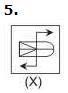Solution:
QUESTION: 8

Select the correct mirror-image of the Figure (X) from amongst the given alternatives.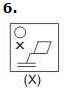Solution:
QUESTION: 9

Each of the following questions consists of five Problem figures marked 1,2,3,4 and 5 followed by five Answer figures marked A,B,C,D and E . Select a figure from the Answer figures which will continue the same series as given in the Problem figures.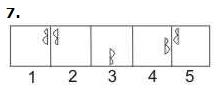Solution:

Explanation : The symbol gets vertically inverted and laterally inverted alternately. It also moves in ACW direction through distances equal to two half-sides (of square boundary) and three half-sides alternately.

QUESTION: 10